## Mike on MATLAB GraphicsGraphics & Data Visualization

Note

Mike on MATLAB Graphics has been retired and will not be updated.

# GraphicsSmoothing and AlignVertexCenters

### GraphicsSmoothing and AlignVertexCenters

In R2014b we introduced a new property on the figure named GraphicsSmoothing. When this is set to on, all of the graphics drawn into that figure are drawn using a technique called Multisample antialiasing. This can really improve how the graphics look on a low res monitor.

Let's draw a bunch of random line segments, first with GraphicsSmoothing set to 'off'.

rng default
figure('GraphicsSmoothing','off')

x = randn(2,100);
x(3,:) = nan;
y = randn(2,100);
y(3,:) = nan;
plot(x(:),y(:))
title('GraphicsSmoothing = off')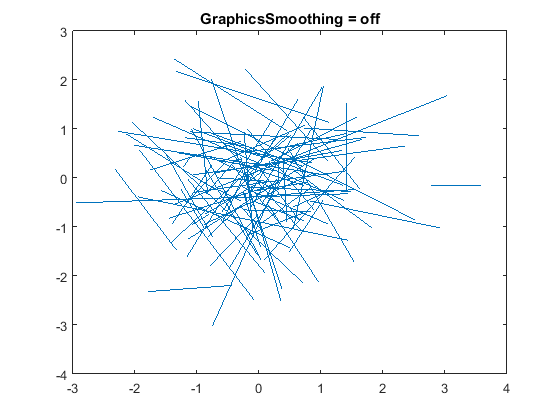And then with GraphicsSmoothing set to 'on'.

set(gcf,'GraphicsSmoothing','on')
title('GraphicsSmoothing = on')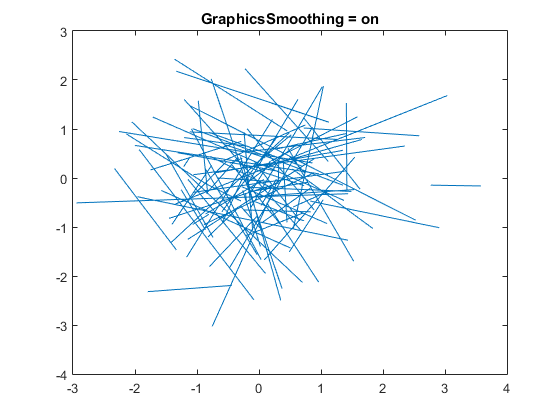The lines that were drawn with GraphicsSmoothing obviously look smoother. If we compare a zoomed in region we can see why.

 GraphicsSmoothing='off' GraphicsSmoothing='on'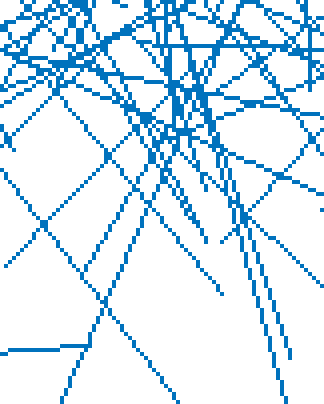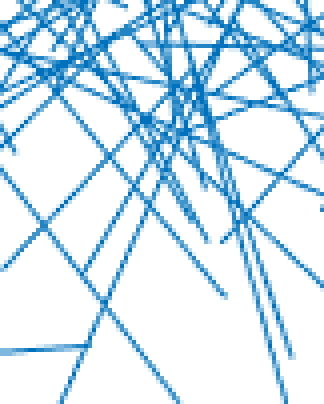We can see that when GraphicsSmoothing is off, the pixels that make up the lines are all the same color. When GraphicsSmoothing is on, the lines are made up of pixels of a number of different colors. These colors are made by mixing the color of the line with the background color. The proportion of the two colors that are mixed together is proportional to how much of that pixel is covered by the line. This tricks your eye into seeing a smooth line instead of one that "jumps" from pixel to pixel.

But there is an issue here. Let's start over with a bunch of vertical lines.

close
figure('GraphicsSmoothing','off')
x = repmat(1:75,[3 1]);
y = repmat([-1; 1; nan],[1 75]);
h = line(x(:),y(:));
ylim([-4 4])
title('GraphicsSmoothing = off')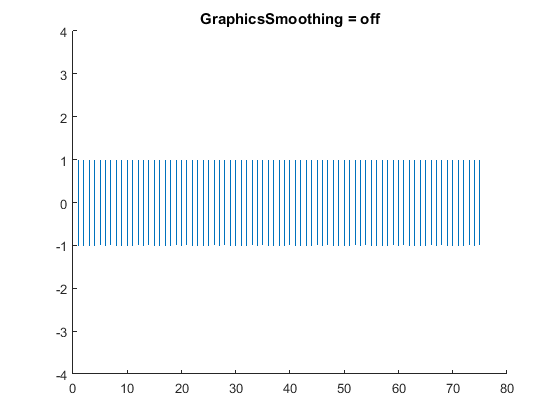When we turn on GraphicsSmoothing this time, we'll notice that some of the lines look darker than others.

set(gcf,'GraphicsSmoothing','on')
title('GraphicsSmoothing = on')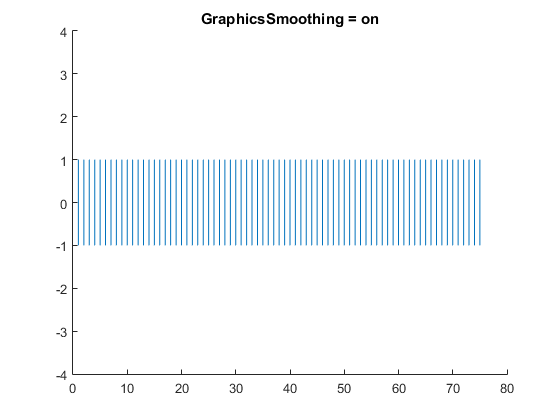This is happening because some of the lines go exactly through a column of pixels and other lines fall in between two columns. The ones that fall in between set two pixels in each row to a light shade, while the ones that go exactly through a column set one pixel in the row to a dark shade.

Your computer has an adjustment in the display settings called gamma correction. If this is not set accurately, then the difference between these two cases can be very visible.

But there is another way to deal with this. The line object has a property named AlignVertexCenters. If we set this to on, then the vertices of the lines will be adjusted so that they land exactly on the pixels. This will make all of the lines look the same.

h.AlignVertexCenters = 'on';
title('AlignVertexCenters = on')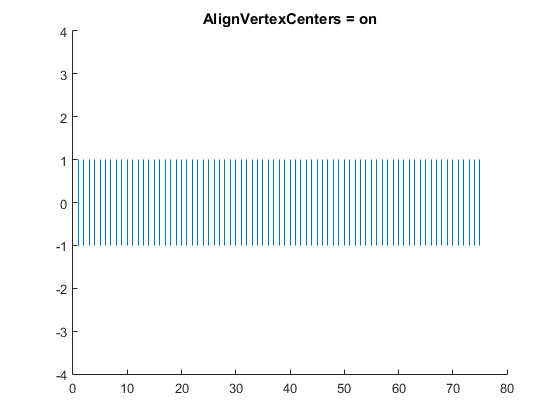So should we always set AlignVertexCenters to on? No, I'm afraid it's not that simple. One thing you'll notice is that the spacing between the lines looks uneven in the GraphicsSmoothing=off and AlignVertexCenter=on cases. That's because the distance between the centers of the lines is a little more than 5 pixels. This means that when they get snapped to the pixel grid, you get a mix of 4 pixel gaps and 5 pixel gaps. In the GraphicsSmoothing=on case thing don't look at "gap toothed" because the shading fills in the edges of the wider gaps.

But there's another important reason that you might not want to use AlignVertexCenters. Let's look at another case.

clf
ang = linspace(0,2*pi,30)';
x = repmat([cos(ang); nan],[1 12]) + repmat(3*(1:12),[31 1]);
y = repmat([sin(ang); nan],[1 12]);
h = line(x(:),y(:));
axis equal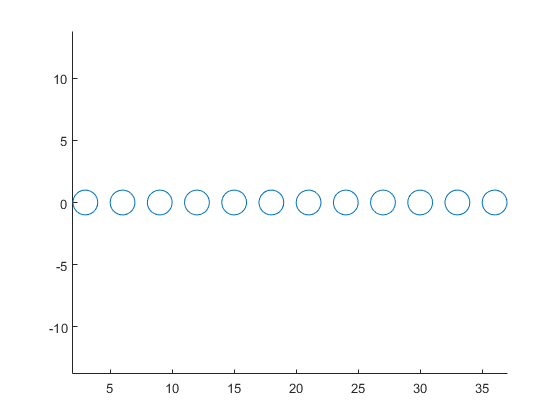Those circles look nice and round, but if we turn AlignVertexCenters, they don't look as good, do they?

h.AlignVertexCenters = 'on';
title('AlignVertexCenters = on')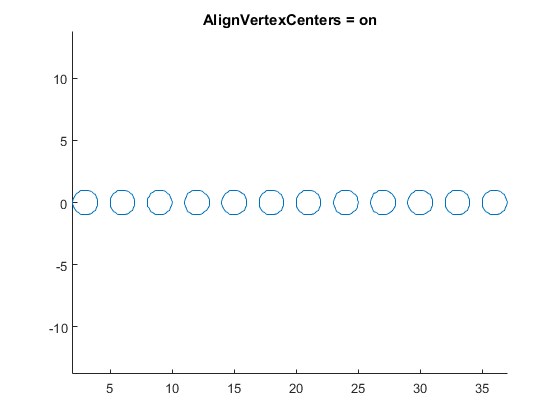For smooth shapes like these circles, we don't want to use AlignVertexCenters because distorts the shape by moving each of the vertices to the nearest pixel.

In general, you only want to use AlignVertexCenters when you're drawing something that's only made up of a relatively small number of horizontal or vertical lines.

Antialiasing is a particularly interesting corner of computer graphics, and I'll be talking about it more in future posts. Do you have any questions about it that you'd like me to address?

Published with MATLAB® R2015a

|